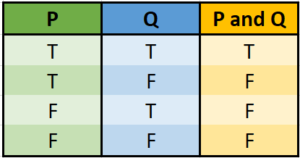# Propositional Calculus & Predicate: Simple Definition

## What is Propositional Calculus?

Propositional calculus (sometimes called sentential calculus) is a simplified version of symbolic logic; It is a way to analyze truth relationships between compound propositions and their individual parts (Kahn, 2007).

The calculus involves a series of simple statements connected by propositional connectives like:

• and (conjunction),
• not (negation),
• or (disjunction),
• if / then / thus (conditional).

You can think of these as being roughly equivalent to basic math operations on numbers (e.g. addition, subtraction, division,…). Only here, instead of numbers, we’re working with propositions (also called statements).

## Classical Propositional CalculusA truth table for a conjunction (“and”) in propositional calculus.In classical propositional calculus, statements can only take on two values: true or false, but not both at the same time. For example, all of the following are statements:

• Albany is the capitol of New York (True),
• King Henry VIII had sixteen wives (False).

The following are not propositional statements, because they don’t have a clear true/false answer, or have a subjective answer:

• He is a good swimmer,
• Banksy is a great artist,

This calculi forms the basis of the majority of logical-mathematical theories; Many complex problems can be reduced to a simple propositional calculus statements, making them easier to solve (Hazelwinkel, 2013). Predicate Calculus is a more complex version, allowing relations, quantifiers, and functions of any number of variables (Goldmakher, 2020).

## What is Predicate Calculus?

The object of predicate calculus, a generalization of propositional calculus, is to identify individuals, along with their predicates and properties.
As an example, the following argument cannot be expressed using propositional calculus, but it can be expressed with predicate calculus (ari, n.d.):

1. All dogs have tails.
2. Butch is a dog.

From these sentences, you could conclude that Butch (the individual) has a tail. “All dogs have tails” is an example of a predicate—used to describe properties or relationships between individuals or objects. “Dogs” and “Tails” are the terms of the statement, which have the same role in logic as nouns and pronouns in language.

## References

Chang, C. & Lee, R. (1997). Symbolic Logic and Mechanical Theorem Proving. New York: Academic Press.
Cundy, H. & Rollett, A. (1989). Mathematical Models, 3rd ed. Stradbroke, England: Tarquin Pub., pp. 254-255.
Goldmakher, L. (2020). Propositional and Predicate Calculus.
Hazelwinkel, M. (2013). Encyclopaedia of Mathematics: Monge—Ampère Equation — Rings and Algebras. Springer.
Kahn, P. (2007). Math 304. Symbolic Logic I: The Propositional Calculus. Retrieved October 29, 2020 from: http://pi.math.cornell.edu/~kahn/SymbLog_PropCalc.pdf
Kari, L/ (n.d.). Predicate Calculus. Retrieved August 4, 2021 from: https://www.csd.uwo.ca/~lkari/logic14.pdf

CITE THIS AS:
Stephanie Glen. "Propositional Calculus & Predicate: Simple Definition" From StatisticsHowTo.com: Elementary Statistics for the rest of us! https://www.statisticshowto.com/propositional-calculus/